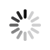#### Welcome!

×Forgot password?
Go back to Challenges list

Challenge

## The Overlapping Squares

In this challenge you will receive the coordinates of two squares and you need to determine if there is an overlapping region formed by those two squares and the area of that region.###### Input Format
Each test case contains the four coordinates of the first square followed by the four coordinates of second square in the following format: "(0,-2) (2,-2) (2,-4) (0,-4) (1,-3) (3,-3) (3,-5) (1,-5)"

###### Output Format
For each test case output the area of the overlapping region or zero if there is no overlap.

###### Sample Inputs
`(0,-2) (2,-2) (2,-4) (0,-4) (1,-3) (3,-3) (3,-5) (1,-5)`

`1`

`512M`